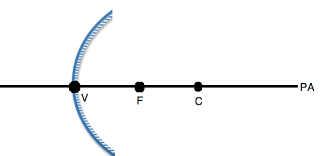# An object is placed at a distance of 40cm in front of a convex mirror of radius of curvature 40cm. List four characteristics of the image formed by the mirror.

Given: An object is placed at a distance of 40cm in front of a convex mirror of radius of curvature of 40cmSo, $u = - 40 \ cm, \ R = + 40 \ cm$

Focal length $f = \frac{R}{2} = \frac{40}{2}$

Using mirror formula:

$\frac{1}{v}+\frac{1}{u}=\frac{1}{f}$

We can rewrite it as: $\frac{1}{v}=\frac{1}{f}-\frac{1}{u}$

Therefore, $\frac{1}{v}=\frac{1}{20}-\frac{1}{-40}=\frac{3}{40}$

So, $v=\frac{40}{3} \mathrm{cm}$

We also know magnification, $m = \frac{-v}{u}$

$m = \frac{-\frac{40}{3}}{- 40}$

So, $m = \frac{1}{3}$

From the values of $v$ and $m$, we can identify the following characteristics of the image:

• The image is virtual as $v$ is positive
• The image is erect as $m$ is positive
• The image is diminished as $m • The image is between focus and pole as$ 0

Updated on: 10-Oct-2022

609 Views